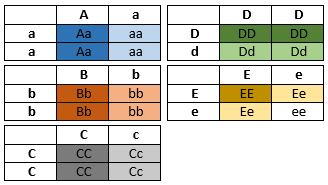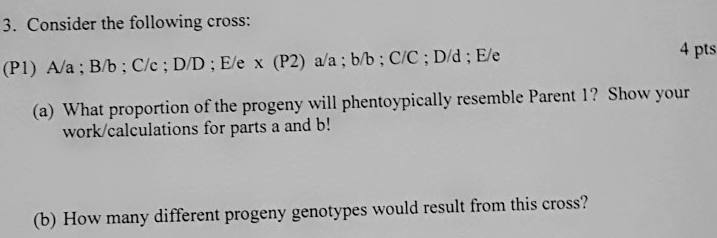# Problem: Consider the following cross: (P1) A/a ; B/b ; C/c ; D/D ; E/e x (P2) a/a ; b;b ; C/C ; D/d ; E/e(a) What proportion of the progeny will phenotypically resemble Parent 1? Show your calculations.(b) How many different progeny genotypes would result from this cross?

###### FREE Expert Solution

The Punnet squares for each gene of this cross is shown below:Since we are dealing with five genes, it would be easier to visualize the chances when we compute for them separately.###### Problem Details

Consider the following cross: (P1) A/a ; B/b ; C/c ; D/D ; E/e x (P2) a/a ; b;b ; C/C ; D/d ; E/e

(a) What proportion of the progeny will phenotypically resemble Parent 1? Show your calculations.

(b) How many different progeny genotypes would result from this cross?